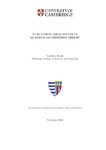## Functional inequalities in quantum information theory

Rouzé, Cambyse
##### DOIThesis
##### Abstract

Functional inequalities constitute a very powerful toolkit in studying various problems arising in classical information theory, statistics and many-body systems. Extensions of these tools to the noncommutative setting have been introduced in the beginning of the 90's in order to study the asymptotic properties of certain quantum Markovian evolutions. In this thesis, we study various extensions and problems arising from the specific noncommutative nature of such processes.

The first logarithmic Sobolev inequality to be proved, due to Gross, was for the Ornstein Uhlenbeck semigroup, that is the Brownian motion with friction on the real line. The generalization of this result to the quantum Ornstein Uhlenbeck semigroup was found very recently by Carlen and Maas, and de Palma and Huber by means of different techniques. The latter proof consists of a quantum generalization of the so-called entropy power inequality. Here, we consider another possible version of the entropy power inequality and use it to derive asymptotic properties of the frictionless quantum Brownian motion.

The proof of Carlen and Maas discussed in the previous paragraph relies on their new quantum extension of the classical notion of displacement convexity. This is classically known to imply most of the usual functional inequalities such as the modified logarithmic Sobolev inequality and Poincaré's inequality. Here, we further study the framework introduced by Carlen and Maas. In particular, we show how displacement convexity implies quantum functional and transportation cost inequalities. The latter are then used to derive certain concentration inequalities of quantum states in the spirit of Bobkov and Goetze. These concentration inequalities are used in order to derive finite sample size bounds for the task of quantum parameter estimation.

The main advantage of classical logarithmic Sobolev inequalities over other methods resides in their tensorization property: the strong log-Sobolev constant of the product of independent Markovian evolutions is equal to the maximum over the set of strong log-Sobolev constants of the individual evolutions. However, this property is strongly believed to fail in the non-commutative case, due to the non-multiplicativity of noncommutative Lp to Lq norms. In this thesis, we show tensorization of the logarithmic Sobolev constants for the simplest quantum Markov semigroup, namely the generalized depolarizing semigroup. Moreover, we consider a new general method to overcome the issue of tensorization for general primitive quantum Markov semigroups by looking at their contractivity properties under the completely bounded Lp to Lq norms. This method was first investigated in the restricted case of unital semigroups by Beigi and King.

Noncommutative functional inequalities considered in the present literature only deal with primitive quantum Markovian semigroups which model memoryless irreversible dynamics converging to a specific faithful state. However, quantum Markov semigroups can in general display a much richer behavior referred to as decoherence: In particular, under some mild conditions, any such semigroup is known to converge to an algebra of observables which effectively evolve unitarily. Here, we introduce the concept of a decoherence-free logarithmic Sobolev inequality, and the related notion of hypercontractivity of the associated evolution, to study the decoherence rate of non-primitive quantum Markov semigroups. Moreover, we utilize the transference method recently introduced by Gao, Junge and LaRacuente, in order to find decoherence times associated to a class of decoherent Markovian evolutions of great importance in the field of quantum error protection, namely collective decoherence semigroups.

Finally, we develop the notion of quantum reverse hypercontractivity, first introduced by Cubitt, Kastoryano, Montanaro and Temme in the unital case, and apply it in conjunction with the tensorization of the modified logarithmic Sobolev inequality for the generalized depolarizing semigroup in order to find strong converse rates in quantum hypothesis testing and for the classical capacity of classical-quantum channels. Moreover, the transference method also allows us to find strong converse bounds on the various capacities of quantum Markovian evolutions.

2018-11-15